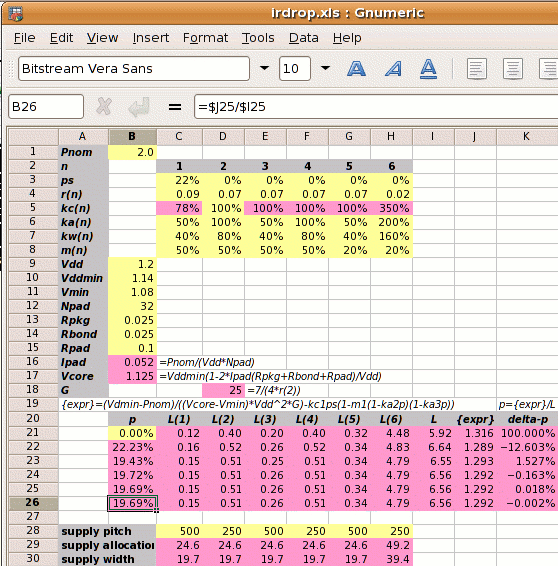The horizontal power straps have half the allocation (or twice the pitch) of vertical ones; metal resistivities are different; core power consumption is 2W with 32 core Vdd and 32 core Vss pads; 30% core is RAM blocked to metal-4; 20% is analog blocked to all layers. It is the same as a previous example but with RAM and analog blocks.

Step 1: Calculate Ipad and Vcore:

2⁄(1.2×32) = 0.052A
Vcore =
1.14×(1−2×0.052×(0.025+0.025+0.1)⁄1.2
1.125V

Step 2: Calculate the reference power supply conductance G:

G =
 7 4×r2

7 ⁄ (4 × 0.07) =  25 mhos

Step 3 is to set out the values of kan, kwn, kcn and mn for each metal layer, and use these to calculate the value of L.

metal layer 1 2 3 4 5 6
kan  50% 100%  50% 100%  50% 200%
power metal allocated coefficient
kwn  80%  80%  80%  80%  80%  80%
power metal used coefficient
kcn  78%¹ 100% 100% 100% 100% 350%²
conductivity coefficient
mn  50%  50%  50%  50%  20%  20%
core area blocked
¹78%=.07/.09; ²350%=.07/.02

The value of L depends on p which we don't know. We iterate to the solution and use p=0 for the first estimate.

 L = kw1kc1(1-ps)(1-m1(1-ka2p)(1-ka3p))+ kw2kc2(1-m2(1-ka2p)(1-ka3p))+ kw3kc3(1-m3(1-ka2p)(1-ka3p))+ kw4kc4(1-m4(1-ka2p)(1-ka3p))+ kw5kc5(1-m5(1-ka2p)(1-ka3p))+ kw6kc6(1-m6(1-ka2p)(1-ka3p)) = ( 0.12 + 0.4 + 0.2 + 0.4 + 0.32 + 4.48 ) = 5.92

Step 4: Calculate the power strap allocation percentage p. The solution must be iterated, and the calculation below shows the first iteration.

 m1′ = m1×(1-ka2p)(1-ka3p)
p =
 { Vddmin×Pnom −kc1×ps(1-m1′) } × 1 (Vcore−Vmin)×Vdd2×G L
 { 1.14×2 −0.78×0.22×(1-0.5) } × 1 (1.125−1.08)×1.22×25 5.92
(1.403−0.086)×0.169 = 22.23%

As shown on the right, a spreadsheet can be used to iterate to the answer of p=19.69%.

If the designer sets the power strap pitch, then the supply allocation for each metal layer n is pitch×kan×p⁄2 and the supply width is pitch×kwn×p⁄2. An example is shown in the spreadsheet on the right where we have chosen a vertical power strap pitch of 250µm.

Step 5: Calculate the new core size. If the initial core size estimate without power straps is x, then with power straps the core size becomes x

 x′ = x = x = x+17.53% √(((1−ka2p)(1−ka3p)) √(0.8031×0.9015)

The value 17.53% is called the IR Drop Adder.

The presence of the fixed blocks has increased the percentage of metal which must be allocated to power straps from 14.92% to 19.69%, an increase of 32%.

Design Attribute Value
Pnom core power consumption 2W
ps fraction of metal-1 in the standard cells used for power supplies 22% (for vsclib)
r1 resistivity of metal layer 1 in ohms per square 0.09Ω per sq.
r2-5 resistivity of metal layers 2-5 in ohms per square 0.07Ω per sq.
r6 resistivity of metal layer 6 in ohms per square 0.02Ω per sq.
ka1,3,5
 user defined   ratio of metal layers 1,3,5 allocated to power metal-2 allocated to power
50%
ka4
 user defined   ratio of metal layer 4 allocated to power metal-2 allocated to power
100%
ka6
 user defined   ratio of metal layer 6 allocated to power metal-2 allocated to power
200%
m1-4 metal layers 1-4 blocked to power straps 50%
m5-6 metal layers 5-6 blocked to power straps 20%
Vdd the nominal supply voltage 1.2V
Vddmin the minimum supply voltage, 5% less than nominal 1.14V
Vmin the desired voltage at the centre of the die, 10% less than the nominal 1.08V
Rpkg the resistance of the package leadframe 25mΩ
Rbond the resistance of the bond wire 25mΩ